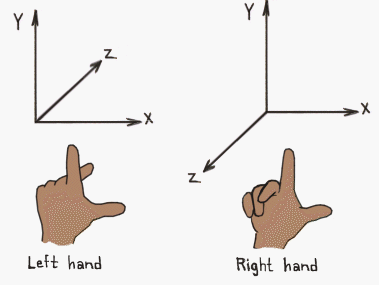top of page
Search
• Lingheng Tao

This blog writes about some basic maths that will be used in the implementation of shaders.

### §1.1 Coordinate System

Some concepts:

1. Orthonormal basis: 3 basis vectors that are perp to each other; the length of each is 1.

2. Orthogonal basis: 3 basis vectors that are perp to each other, whose lengths are not necessarily 1.

3. Handed Coordinate Space: left-handed c.s. vs. right-handed c.s. ALWAYS, your thumb is pointing to +x, and your index finger is pointing to +y.4. Positive rotation: in the handed system, use your corresponding hand; thumbs up along the direction of the axis, then the rotation of the remaining 4 fingers defines the positive direction of rotation.

Note: Unity uses the left-handed coordinate system, where right, up, and forward corresponds to +x, +y, and +z. i.e. the camera is facing to the negative direction of z-axis.

### §1.2 Vector

Some concepts:

1. A 3D vector has form a = (x, y, z), 0 = (0,0,0).

2. Scalar times Vector

3. ba = b(x, y, z) = (bx, by, bz).

4. b can be any scalar.

5. Vector plus Vector

6. a = (a1, a2, a3), b = (b1, b2, b3), a+b = (a1+b1, a2+b2, a3+b3)

7. a - b = a + (-1)b.

8. Magnitude

9. |a| = sqrt(x^2 + y^2 + z^2).

10. Normalized vector ^v = v/|v|, where v is not 0. The normalized vector always has length(magnitude) 1.

11. Dot product

12. a = (a1, a2, a3), b = (b1, b2, b3), a·b = (a1b1, a2b2, a3b3)

13. a·b = |a||b|cos<a,b>, where <a,b> is the angle between a and b.

14. Geometric understanding: ^a · b is the signed projection of b on ^a.

15. Cross product

16. a = (a1, a2, a3), b = (b1, b2, b3), a×b = (a2b3 - a3b2, a3b1 - a1b3, a1b2-a2b1).

17. a × b = -b × a.

18. |a×b| = |a||b|sin<a, b>. It is the area of the parallelogram constructed by the 2 vectors.